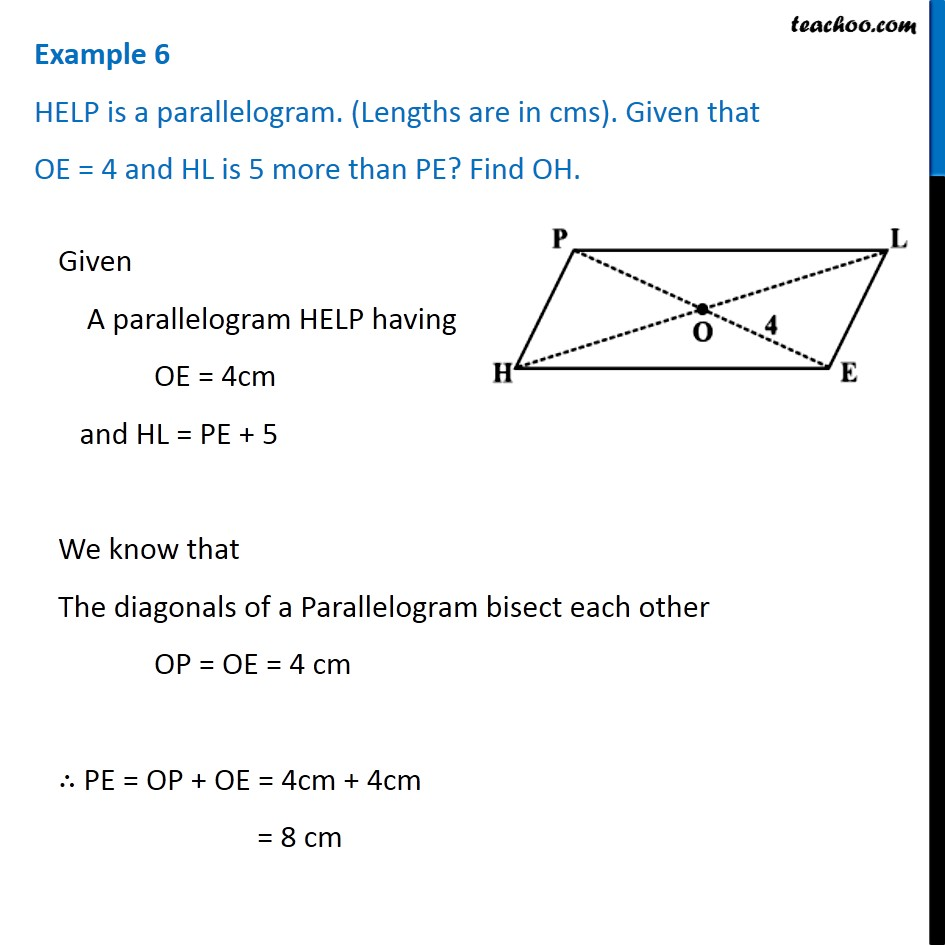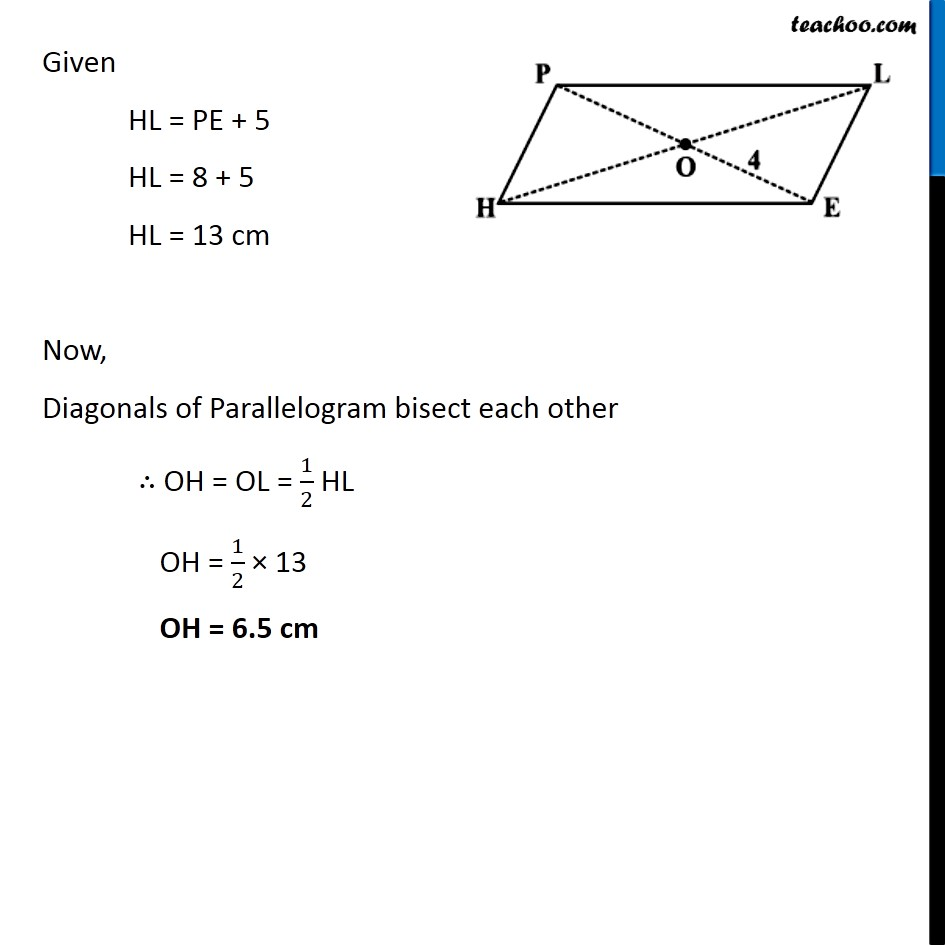Examples

Chapter 3 Class 8 Understanding Quadrilaterals
Serial order wiseLearn in your speed, with individual attention - Teachoo Maths 1-on-1 Class

### Transcript

Example 6 HELP is a parallelogram. (Lengths are in cms). Given that OE = 4 and HL is 5 more than PE? Find OH.Given A parallelogram HELP having OE = 4cm and HL = PE + 5 We know that The diagonals of a Parallelogram bisect each other OP = OE = 4 cm ∴ PE = OP + OE = 4cm + 4cm = 8 cm Given HL = PE + 5 HL = 8 + 5 HL = 13 cm Now, Diagonals of Parallelogram bisect each other ∴ OH = OL = 1/2 HL OH = 1/2 × 13 OH = 6.5 cm